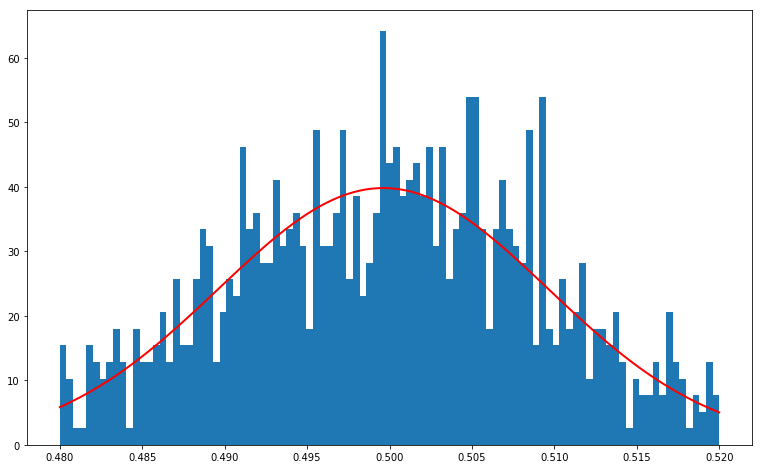# Some basics around probabilities

## basics of probability theory¶

In the context of a course in Computational Neuroscience, I am teaching a basic introduction in Probabilities, Bayes and the Free-energy principle.

Let's learn to use probabilities in practice by generating some "synthetic data", that is by using the computer's number generator. 2017-03-13_NeuroComp_FEP

Let's begin with a dice and the numpy.random library which provides with a wide range of function to handle random variables:

### dice¶

the numpy library is a module

In :
import numpy as np
np

Out:
<module 'numpy' from '/usr/local/lib/python3.6/site-packages/numpy/__init__.py'>

from which you can use numpy.random

In :
np.random

Out:
<module 'numpy.random' from '/usr/local/lib/python3.6/site-packages/numpy/random/__init__.py'>
help(np.random.logistic)
In :
help(np.random.randint)

Help on built-in function randint:

randint(...) method of mtrand.RandomState instance
randint(low, high=None, size=None, dtype='l')

Return random integers from low (inclusive) to high (exclusive).

Return random integers from the "discrete uniform" distribution of
the specified dtype in the "half-open" interval [low, high). If
high is None (the default), then results are from [0, low).

Parameters
----------
low : int
Lowest (signed) integer to be drawn from the distribution (unless
high=None, in which case this parameter is one above the
*highest* such integer).
high : int, optional
If provided, one above the largest (signed) integer to be drawn
from the distribution (see above for behavior if high=None).
size : int or tuple of ints, optional
Output shape.  If the given shape is, e.g., (m, n, k), then
m * n * k samples are drawn.  Default is None, in which case a
single value is returned.
dtype : dtype, optional
Desired dtype of the result. All dtypes are determined by their
name, i.e., 'int64', 'int', etc, so byteorder is not available
and a specific precision may have different C types depending
on the platform. The default value is 'np.int'.

Returns
-------
out : int or ndarray of ints
size-shaped array of random integers from the appropriate
distribution, or a single such random int if size not provided.

--------
random.random_integers : similar to randint, only for the closed
interval [low, high], and 1 is the lowest value if high is
omitted. In particular, this other one is the one to use to generate
uniformly distributed discrete non-integers.

Examples
--------
>>> np.random.randint(2, size=10)
array([1, 0, 0, 0, 1, 1, 0, 0, 1, 0])
>>> np.random.randint(1, size=10)
array([0, 0, 0, 0, 0, 0, 0, 0, 0, 0])

Generate a 2 x 4 array of ints between 0 and 4, inclusive:

>>> np.random.randint(5, size=(2, 4))
array([[4, 0, 2, 1],
[3, 2, 2, 0]])



Let's draw a cubic dice 10 times:

In :
np.random.randint(6, size=10)

Out:
array([2, 4, 2, 2, 4, 3, 4, 5, 4, 0])

### a note on RNGs¶

On a computer, randomness is (possibly) deterministic !

In :
np.random.randint(6, size=10)

Out:
array([5, 2, 5, 5, 3, 3, 0, 5, 4, 2])
In :
np.random.seed(42)
np.random.randint(6, size=10)

Out:
array([3, 4, 2, 4, 4, 1, 2, 2, 2, 4])
In :
np.random.seed(43)
np.random.randint(6, size=10)

Out:
array([4, 0, 1, 5, 2, 0, 3, 1, 3, 3])
In :
np.random.seed(42)
np.random.randint(6, size=10)

Out:
array([3, 4, 2, 4, 4, 1, 2, 2, 2, 4])
In :
np.random.seed(None)
np.random.randint(6, size=10)

Out:
array([4, 1, 3, 1, 4, 3, 0, 0, 1, 3])

### coin¶

In :
result = np.random.randint(2, size=10)
print(result)

[1 0 0 0 0 1 0 0 1 1]

In :
result.mean(), result.std(), result.var()

Out:
(0.4, 0.4898979485566356, 0.24)
In :
N = 1000
result = np.random.randint(2, size=N)
print('Mean ', result.mean(), ', std ', result.std())

Mean  0.515 , std  0.49977494935220584

In :
N = 1000
N_trials = 100
result = np.random.randint(2, size=(N, N_trials))
print('Mean ', result.mean(axis=0), ', std ', result.mean(axis=0).std())

Mean  [0.487 0.471 0.501 0.476 0.479 0.505 0.485 0.491 0.495 0.479 0.502 0.516
0.474 0.523 0.48  0.535 0.473 0.502 0.525 0.484 0.517 0.498 0.471 0.495
0.481 0.469 0.483 0.523 0.486 0.506 0.498 0.515 0.497 0.51  0.491 0.487
0.532 0.485 0.509 0.512 0.498 0.481 0.501 0.509 0.509 0.509 0.502 0.507
0.482 0.526 0.476 0.501 0.483 0.483 0.512 0.51  0.497 0.482 0.511 0.48
0.473 0.5   0.485 0.519 0.488 0.513 0.536 0.476 0.487 0.484 0.5   0.502
0.505 0.514 0.486 0.538 0.485 0.51  0.512 0.504 0.496 0.502 0.475 0.5
0.495 0.486 0.465 0.519 0.496 0.534 0.473 0.5   0.519 0.493 0.501 0.482
0.524 0.51  0.53  0.503] , std  0.017229541491287588

In :
print('Grand average=', result.mean())

Grand average= 0.49827

In :
%matplotlib inline
import matplotlib.pyplot as plt

In :
N = 1000
N_trials = 100
result = np.random.randint(2, size=(N, N_trials))
print('Mean ', result.mean(axis=0), ', std ', result.mean(axis=0).std())
fig, ax = plt.subplots(figsize=(13, 8))
ax.hist(result.mean(axis=0), bins=np.linspace(0, 1, 100));

Mean  [0.521 0.511 0.501 0.503 0.472 0.514 0.499 0.522 0.498 0.491 0.517 0.509
0.495 0.521 0.514 0.508 0.523 0.493 0.516 0.509 0.5   0.504 0.478 0.492
0.473 0.517 0.493 0.48  0.485 0.513 0.536 0.51  0.508 0.505 0.532 0.482
0.484 0.505 0.485 0.497 0.495 0.523 0.497 0.508 0.489 0.458 0.524 0.515
0.508 0.52  0.519 0.497 0.474 0.528 0.507 0.512 0.497 0.494 0.475 0.475
0.477 0.523 0.501 0.509 0.506 0.51  0.508 0.492 0.483 0.507 0.501 0.486
0.507 0.482 0.482 0.503 0.5   0.5   0.509 0.509 0.545 0.505 0.472 0.505
0.476 0.518 0.499 0.487 0.521 0.516 0.491 0.505 0.501 0.489 0.495 0.492
0.504 0.507 0.499 0.481] , std  0.015810894977830964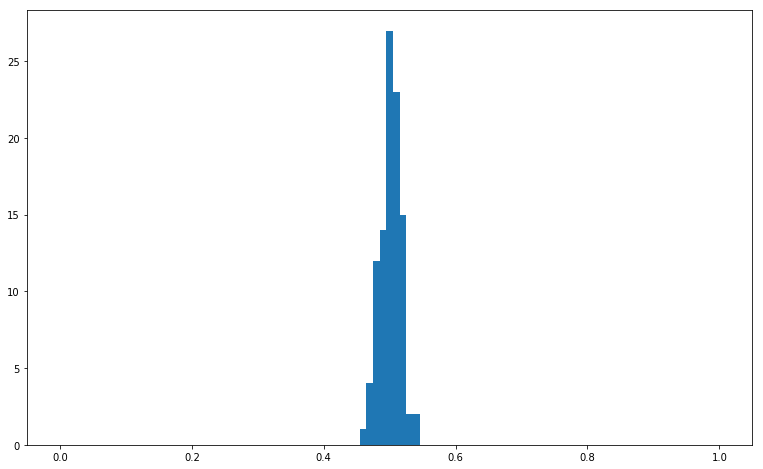In :
N = 100
N_trials = 1000
result = np.random.randint(2, size=(N, N_trials))
print('Mean ', result.mean(axis=0), ', std ', result.mean(axis=0).std())
fig, ax = plt.subplots(figsize=(13, 8))
ax.hist(result.mean(axis=0), bins=np.linspace(0, 1, 100));

Mean  [0.49 0.51 0.52 0.52 0.47 0.53 0.51 0.55 0.44 0.53 0.47 0.54 0.5  0.49
0.52 0.6  0.58 0.5  0.51 0.51 0.44 0.5  0.44 0.49 0.47 0.43 0.53 0.47
0.5  0.45 0.52 0.44 0.45 0.49 0.48 0.55 0.45 0.58 0.51 0.58 0.56 0.61
0.58 0.46 0.53 0.55 0.45 0.53 0.51 0.47 0.44 0.45 0.51 0.42 0.44 0.61
0.48 0.4  0.54 0.55 0.42 0.42 0.51 0.49 0.54 0.52 0.5  0.46 0.43 0.45
0.5  0.45 0.53 0.48 0.49 0.48 0.51 0.53 0.46 0.47 0.46 0.45 0.56 0.49
0.45 0.51 0.55 0.47 0.53 0.58 0.54 0.48 0.57 0.49 0.48 0.48 0.45 0.53
0.46 0.38 0.52 0.47 0.48 0.5  0.5  0.43 0.45 0.48 0.51 0.48 0.45 0.41
0.43 0.48 0.55 0.45 0.54 0.45 0.35 0.56 0.5  0.61 0.61 0.57 0.46 0.55
0.49 0.57 0.54 0.52 0.5  0.52 0.56 0.49 0.51 0.5  0.49 0.59 0.56 0.47
0.49 0.54 0.52 0.49 0.42 0.45 0.5  0.48 0.5  0.49 0.42 0.52 0.51 0.46
0.49 0.46 0.57 0.49 0.53 0.47 0.53 0.51 0.57 0.53 0.47 0.52 0.52 0.39
0.5  0.51 0.47 0.49 0.42 0.58 0.52 0.54 0.43 0.41 0.57 0.44 0.55 0.5
0.49 0.58 0.46 0.55 0.49 0.44 0.36 0.52 0.45 0.44 0.44 0.4  0.58 0.56
0.47 0.51 0.46 0.52 0.58 0.53 0.48 0.47 0.4  0.55 0.5  0.43 0.52 0.52
0.47 0.67 0.48 0.54 0.54 0.56 0.54 0.6  0.55 0.52 0.45 0.55 0.55 0.49
0.56 0.55 0.5  0.46 0.51 0.54 0.58 0.48 0.51 0.5  0.47 0.49 0.54 0.49
0.49 0.52 0.54 0.58 0.57 0.51 0.45 0.5  0.54 0.57 0.58 0.59 0.45 0.5
0.51 0.48 0.46 0.5  0.53 0.49 0.5  0.47 0.55 0.51 0.52 0.52 0.53 0.44
0.43 0.48 0.44 0.43 0.48 0.41 0.55 0.39 0.46 0.52 0.49 0.53 0.44 0.52
0.59 0.48 0.5  0.44 0.48 0.49 0.54 0.5  0.49 0.43 0.5  0.49 0.5  0.47
0.44 0.46 0.49 0.5  0.4  0.51 0.48 0.59 0.56 0.46 0.5  0.42 0.45 0.51
0.47 0.52 0.54 0.53 0.48 0.48 0.41 0.54 0.43 0.48 0.38 0.47 0.46 0.53
0.59 0.6  0.5  0.54 0.51 0.42 0.4  0.49 0.42 0.41 0.42 0.52 0.45 0.48
0.51 0.49 0.41 0.51 0.49 0.47 0.56 0.5  0.41 0.47 0.5  0.55 0.58 0.49
0.51 0.41 0.51 0.51 0.4  0.57 0.46 0.53 0.58 0.48 0.54 0.46 0.49 0.55
0.43 0.5  0.43 0.56 0.48 0.49 0.53 0.47 0.55 0.53 0.48 0.5  0.56 0.55
0.48 0.49 0.47 0.5  0.57 0.58 0.52 0.51 0.52 0.42 0.5  0.54 0.59 0.43
0.5  0.5  0.53 0.5  0.58 0.47 0.51 0.56 0.55 0.45 0.38 0.55 0.53 0.54
0.57 0.5  0.49 0.49 0.48 0.5  0.56 0.44 0.56 0.49 0.59 0.6  0.52 0.53
0.49 0.4  0.45 0.52 0.55 0.45 0.48 0.5  0.44 0.5  0.47 0.57 0.51 0.52
0.49 0.4  0.49 0.47 0.49 0.46 0.5  0.57 0.53 0.45 0.51 0.51 0.55 0.44
0.53 0.49 0.56 0.58 0.48 0.51 0.62 0.46 0.53 0.54 0.5  0.54 0.46 0.46
0.53 0.56 0.63 0.55 0.5  0.49 0.5  0.53 0.53 0.52 0.51 0.49 0.57 0.61
0.51 0.56 0.43 0.47 0.53 0.46 0.47 0.58 0.45 0.52 0.46 0.56 0.46 0.66
0.47 0.41 0.52 0.52 0.48 0.53 0.5  0.58 0.48 0.55 0.58 0.47 0.5  0.55
0.53 0.62 0.51 0.45 0.47 0.53 0.51 0.51 0.42 0.48 0.53 0.51 0.48 0.53
0.46 0.47 0.58 0.55 0.48 0.47 0.52 0.56 0.49 0.46 0.5  0.48 0.54 0.59
0.56 0.47 0.45 0.49 0.39 0.44 0.49 0.55 0.55 0.62 0.41 0.49 0.48 0.54
0.49 0.51 0.52 0.52 0.62 0.43 0.5  0.53 0.43 0.56 0.56 0.54 0.54 0.51
0.45 0.47 0.48 0.44 0.5  0.51 0.44 0.44 0.49 0.49 0.41 0.51 0.48 0.42
0.54 0.5  0.55 0.5  0.43 0.6  0.49 0.47 0.41 0.55 0.45 0.44 0.52 0.44
0.47 0.49 0.54 0.59 0.38 0.53 0.54 0.42 0.5  0.54 0.44 0.5  0.6  0.46
0.44 0.49 0.48 0.54 0.54 0.48 0.46 0.48 0.44 0.54 0.5  0.43 0.59 0.49
0.53 0.56 0.55 0.52 0.52 0.55 0.4  0.51 0.56 0.46 0.55 0.53 0.53 0.48
0.55 0.54 0.55 0.44 0.51 0.51 0.56 0.57 0.57 0.54 0.53 0.49 0.46 0.43
0.46 0.55 0.51 0.45 0.43 0.52 0.44 0.47 0.53 0.57 0.43 0.51 0.42 0.51
0.5  0.55 0.45 0.51 0.58 0.54 0.53 0.54 0.55 0.59 0.53 0.48 0.59 0.49
0.58 0.47 0.49 0.48 0.44 0.46 0.48 0.49 0.41 0.53 0.45 0.5  0.57 0.54
0.53 0.4  0.51 0.5  0.52 0.45 0.44 0.5  0.56 0.58 0.41 0.51 0.4  0.53
0.53 0.6  0.51 0.57 0.56 0.45 0.52 0.6  0.62 0.5  0.5  0.51 0.52 0.48
0.57 0.46 0.42 0.49 0.5  0.55 0.54 0.48 0.57 0.5  0.58 0.44 0.54 0.43
0.39 0.47 0.47 0.36 0.53 0.52 0.48 0.5  0.47 0.47 0.59 0.51 0.54 0.51
0.53 0.57 0.47 0.5  0.47 0.46 0.54 0.45 0.51 0.6  0.51 0.49 0.57 0.56
0.57 0.5  0.51 0.42 0.51 0.5  0.53 0.49 0.56 0.54 0.51 0.55 0.39 0.47
0.51 0.42 0.5  0.54 0.56 0.5  0.52 0.45 0.54 0.48 0.52 0.57 0.38 0.47
0.51 0.58 0.59 0.46 0.35 0.48 0.5  0.45 0.55 0.53 0.47 0.49 0.55 0.56
0.54 0.49 0.42 0.53 0.5  0.52 0.55 0.53 0.52 0.55 0.54 0.56 0.49 0.52
0.48 0.47 0.46 0.48 0.51 0.47 0.51 0.49 0.47 0.63 0.48 0.54 0.49 0.61
0.44 0.42 0.43 0.54 0.53 0.48 0.48 0.49 0.49 0.38 0.47 0.39 0.49 0.51
0.5  0.49 0.51 0.49 0.56 0.44 0.56 0.44 0.46 0.39 0.6  0.49 0.59 0.49
0.57 0.44 0.45 0.53 0.55 0.45 0.48 0.41 0.36 0.52 0.52 0.57 0.45 0.49
0.52 0.5  0.58 0.46 0.54 0.46 0.47 0.53 0.48 0.48 0.53 0.62 0.45 0.61
0.52 0.51 0.54 0.45 0.4  0.43 0.52 0.47 0.43 0.5  0.49 0.48 0.57 0.57
0.49 0.48 0.53 0.52 0.49 0.5  0.51 0.56 0.45 0.51 0.45 0.47 0.49 0.57
0.48 0.53 0.46 0.55 0.46 0.48 0.49 0.5  0.49 0.5  0.51 0.56 0.55 0.53
0.52 0.49 0.48 0.48 0.52 0.49 0.47 0.48 0.48 0.54 0.42 0.45 0.4  0.49
0.46 0.54 0.58 0.48 0.52 0.46 0.46 0.53 0.49 0.44 0.51 0.48 0.6  0.45
0.56 0.53 0.39 0.49 0.52 0.54 0.5  0.55 0.51 0.44 0.49 0.49 0.5  0.6
0.53 0.48 0.56 0.49 0.51 0.6  0.48 0.52 0.52 0.52 0.48 0.57 0.47 0.5
0.41 0.58 0.57 0.51 0.46 0.46 0.47 0.4  0.47 0.65 0.52 0.49 0.55 0.52
0.38 0.41 0.56 0.51 0.52 0.49] , std  0.050859162399709265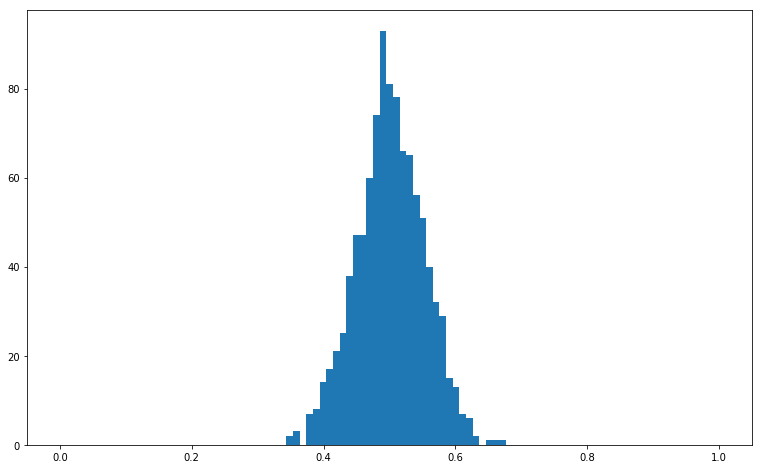In :
N = 10000
N_trials = 10
result = np.random.randint(2, size=(N, N_trials))
print('Mean ', result.mean(axis=0), ', std ', result.mean(axis=0).std())
fig, ax = plt.subplots(figsize=(13, 8))
ax.hist(result.mean(axis=0), bins=np.linspace(0, 1, 100));

Mean  [0.5026 0.4995 0.4993 0.5032 0.4975 0.5013 0.5073 0.4982 0.4968 0.5067] , std  0.0034923344627913297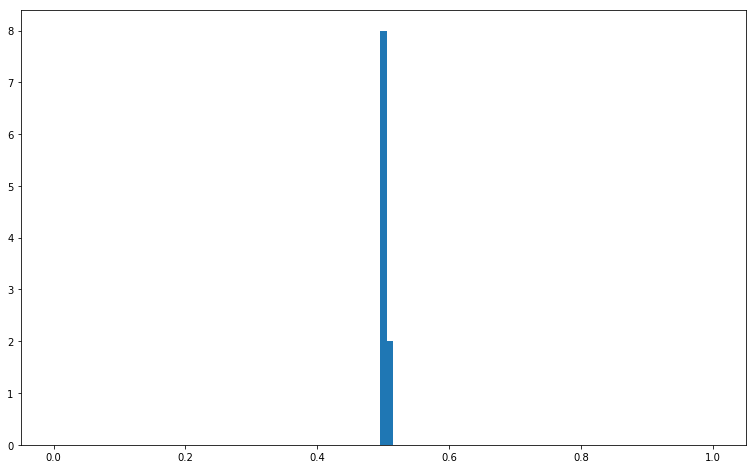In :
N = 10
N_trials = 10000
result = np.random.randint(2, size=(N, N_trials))
print('Mean ', result.mean(axis=0), ', std ', result.mean(axis=0).std())
fig, ax = plt.subplots(figsize=(13, 8))
ax.hist(result.mean(axis=0), bins=np.linspace(0, 1, 100));

Mean  [0.3 0.5 0.5 ... 0.4 0.6 0.8] , std  0.15860277141336465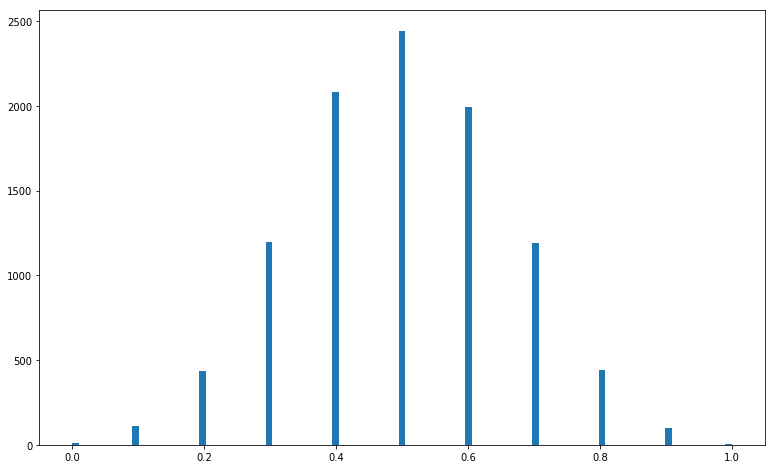### introducing the normal variable: the Gaussian distribution¶

In :
N = 10000
N_trials = 10000
result = np.random.randint(2, size=(N, N_trials))
print('Mean ', result.mean(axis=0), ', std ', result.mean(axis=0).std())
fig, ax = plt.subplots(figsize=(13, 8))
ax.hist(result.mean(axis=0), bins=np.linspace(0.48, .52, 100));

Mean  [0.5037 0.5168 0.5043 ... 0.5018 0.5036 0.5034] , std  0.0049776245637351965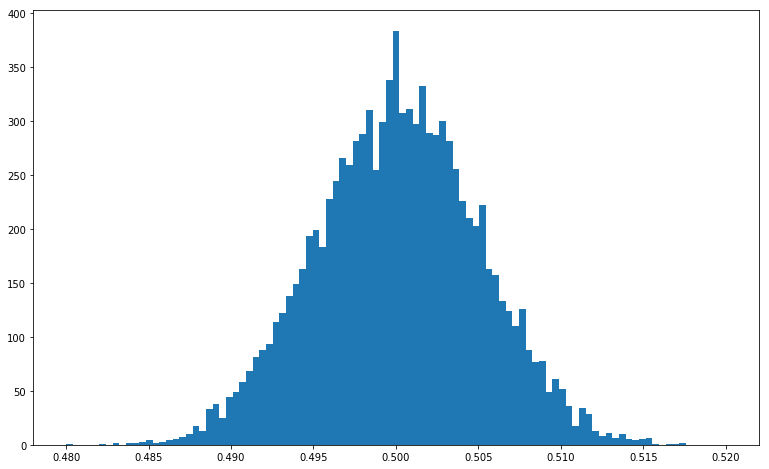Central limit theorem:

Over multiple measurements, the average of an identical random variable converges to a normal law : $$p (x) = \frac {1} { \sqrt{ 2\pi } \sigma} \cdot \exp{(- \frac {1} {2} \cdot \frac {(x - m)^2} {\sigma^2} )}$$

(moreover, we know from this theorem that the asymptotic mean is the mean or the rv and that the variance decreases inversely proportionally with the number of measurements)

In :
mean  = result.mean(axis=0).mean()
std  = result.mean(axis=0).std()
bins = np.linspace(0.48, .52, 100)

fig, ax = plt.subplots(figsize=(13, 8))
ax.hist(result.mean(axis=0), bins=bins, density=True)
ax.plot(bins, 1 / np.sqrt(2*np.pi) / std * np.exp(-.5*(bins-mean)**2/std**2), 'r', lw=2 );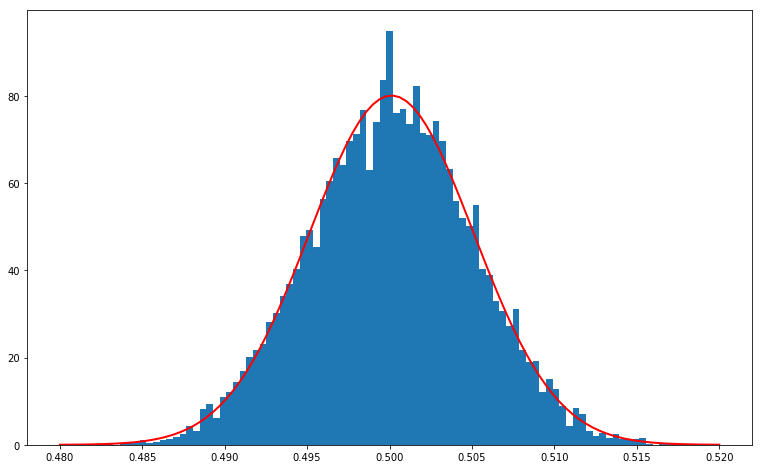The central limit theorem works with all forms of random variables:

In :
N = 10000
N_trials = 1000
result = np.random.rand(N, N_trials)
print(result)

[[0.17776032 0.70153493 0.51930643 ... 0.35664146 0.05377125 0.99831716]
[0.47969204 0.05427134 0.87522712 ... 0.0897827  0.83370048 0.20273843]
[0.8052115  0.62939528 0.12933345 ... 0.31730851 0.62459905 0.56850112]
...
[0.93144067 0.63063853 0.33191078 ... 0.28888445 0.93512079 0.6656451 ]
[0.84540231 0.89137123 0.72865125 ... 0.29466667 0.29699345 0.5264605 ]
[0.29392923 0.51797084 0.41350589 ... 0.30197422 0.91424485 0.94056245]]

In :
mean  = result.mean(axis=0).mean()
std  = result.mean(axis=0).std()
bins = np.linspace(0.48, .52, 100)

fig, ax = plt.subplots(figsize=(13, 8))
ax.hist(result.mean(axis=0), bins=bins, density=True)
ax.plot(bins, 1 / np.sqrt(2*np.pi) / std * np.exp(-.5*(bins-mean)**2/std**2), 'r', lw=2 );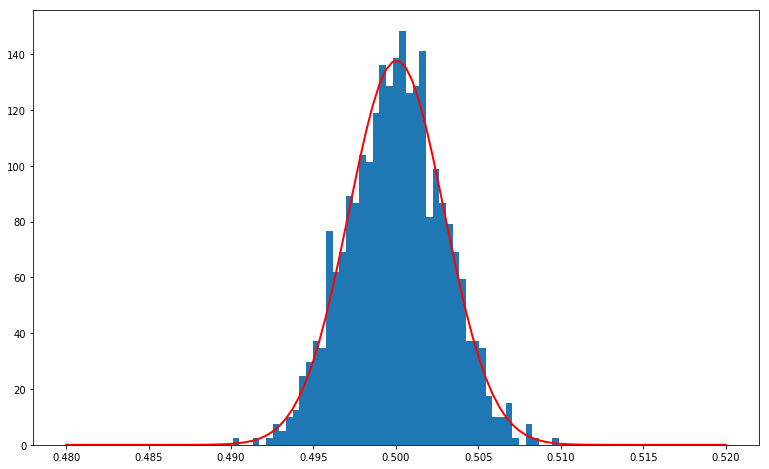In :
N = 10000
N_trials = 1000
result = np.random.randn(N, N_trials) + .5

mean  = result.mean(axis=0).mean()
std  = result.mean(axis=0).std()
bins = np.linspace(0.48, .52, 100)

fig, ax = plt.subplots(figsize=(13, 8))
ax.hist(result.mean(axis=0), bins=bins, density=True)
ax.plot(bins, 1 / np.sqrt(2*np.pi) / std * np.exp(-.5*(bins-mean)**2/std**2), 'r', lw=2 );